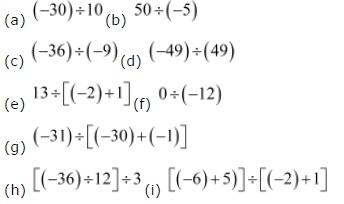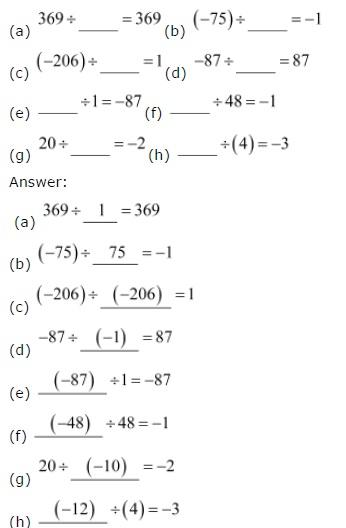# NCERT Solutions class 7 Maths Chapter-1 Exercise 1.4

## Integers Exercise-1.4

NCERT Solutions Class-7 Maths chapter 1 Integer Exercise-1.4 is prepared by academic team of Physics Wallah all the questions of NCERT text book are solved step by step with proper and detail solutions explaining each and every questions. For More and additional questions of CBSE class 7 maths you can go to class 7 maths sections. NCERT class 7 Maths Solutions is the best way to enhanced your mathematics skill. And Physics Wallah practice worksheet & question bank will help you a lot.

### Solutions of Chapter Integeres Exercise-1.4

Question 1:

Evaluate each of the following:(a) (−30) ÷ 10 = −3

(b) 50 ÷ (−5) = −10

(c) (−36) ÷ (−9) = 4

(d) (−49) ÷ 49 = −1

(e) 13 ÷ [−2 + 1] = 13 ÷ [−1] = −13

(f) 0 ÷ (−12) = 0

(g) (−31) ÷ [(−30) + (−1)] = (−31) ÷ (−31) = 1

(h) [(−36) ÷ 12] ÷ 3 = [−3] ÷ 3 = −1

(i) [− 6 + 5] ÷ [− 2 + 1] = (−1) ÷ (−1) = 1

Question 2:

Verify that a ÷ (b + c) ≠ (a ÷ b) + (a ÷ c) for each of the following values of a, b and c.

(a) a = 12, b = −4, c = 2

(b) a = (− 10), b = 1, c = 1Answer:

(a) a = 12, b = −4, c = 2

a ÷ (b + c) = 12 ÷ (− 4 + 2) = 12 ÷ (−2) = −6

(a ÷ b) + (a ÷ c) = [12 ÷ (−4)] + [12 ÷ 2] = −3 + 6 = 3

Hence, a ÷ (b + c) ≠ (a ÷ b) + (a ÷ c)

(b) a = −10, b = 1, c = 1

a ÷ (b + c) = (−10) ÷ (1 + 1) = (−10) ÷ 2 = −5

(a ÷ b) + (a ÷ c) = [(−10) ÷ 1] + [(−10) ÷ 1] = − 10 − 10 = −20

Hence, a ÷ (b + c) ≠ (a ÷ b) + (a ÷ c)

Question 3:

Fill in the blanks:Question 4:

Write five pairs of integers (a, b) such a ÷ b = − 3. One such pair is (6, − 2) because 6 ÷ (− 2) = (− 3).

(i) (3, −1)

Because 3 ÷ (−1) = −3

(ii) (−3, 1)

Because (−3) ÷ 1 = −3

(iii) (9, −3)

Because 9 ÷ (−3) = −3

(iv) (−9, 3)

Because (−9) ÷ 3 = −3

(v) (12, −4)

Because 12 ÷ (−4) = −3

Question 5:

The temperature at 12 noon was 10°C above zero. If it decreases at the rate of 2°C per hour until midnight, at what time would the temperature be 8°C below zero? What would be the temperature at mid-night?

Initial temperature i.e., at 12 noon = 10°C

Change in temperature per hour = −2°C

Temperature at 1:00 PM = 10ºC + (−2ºC) = 8ºC

Temperature at 2:00 PM = 8ºC + (−2ºC) = 6ºC

Temperature at 3:00 PM = 6ºC + (−2ºC) = 4ºC

Temperature at 4:00 PM = 4ºC + (−2ºC) = 2ºC

Temperature at 5:00 PM = 2ºC + (−2ºC) = 0ºC

Temperature at 6:00 PM = 0ºC + (−2ºC) = −2ºC

Temperature at 7:00 PM = −2ºC + (−2ºC) = −4ºC

Temperature at 8:00 PM = −4ºC + (−2ºC) = −6ºC

Temperature at 9:00 PM = −6ºC + (−2ºC) = −8ºC

Therefore, the temperature will be 8°C below zero at 9:00 PM.

It will take 12 hours to be midnight (i.e., 12:00 AM) after 12:00 noon.

Change in temperature in 12 hours = −2°C × 12 = −24ºC

At midnight, the temperature will be = 10 + (−24)

= −14°C

Therefore, the temperature at midnight will be 14ºC below 0.

Question 6:

In a class test (+ 3) marks are given for every correct answer and (−2) marks are given for every incorrect answer and no marks for not attempting any question. (i) Radhika scored 20 marks. If she has got 12 correct answers, how many questions has she attempted incorrectly? (ii) Mohini scores − 5 marks in this test, though she has got 7 correct answers. How many questions has she attempted incorrectly? (iii) Rakesh scores 18 marks by attempting 16 questions. How many questions has he attempted correctly and how many has he attempted incorrectly?

Marks obtained for 1 right answer = +3

Marks obtained for 1 wrong answer = −2

(i) Marks scored by Radhika = 20

Marks obtained for 12 correct answers = 12 × 3 = 36

Marks obtained for incorrect answers = Total score − Marks obtained for 12 correct answers

= 20 − 36 = −16

Marks obtained for 1 wrong answer = −2

Thus, number of incorrect answers = (−16) ÷(−2) = 8

Therefore, she attempted 8 questions wrongly.

(ii) Marks scored by Mohini = −5

Marks obtained for 7 correct answers = 7 × 3 = 21

Marks obtained for incorrect answers = Total score − Marks obtained for 12 correct answers

= − 5 − 21 = −26

Marks obtained for 1 wrong answer = −2

Thus, number of incorrect answers = (−26) ÷(−2) = 13

Therefore, she attempted 13 questions wrongly.

(iii) Total marks scored by Rakesh = 18

Number of questions attempted = 16

⇒(Number of correct answers)(3) + (16 − Number of correct answers)(−2) = 18

⇒(Number of correct answers)(3) + −32 + 2(Number of correct answers) = 18

⇒(Number of correct answers)(5) + −32 = 18

⇒(Number of correct answers)(5) = 18 + 32 = 50

⇒ Number of correct answers = 10

∴Number of incorrect answers = 16 − 10 = 6

∴Total number of correct and incorrect answers scored by Rakesh is 10 and 6 respectively.

Question 7:

An elevator descends into a mine shaft at the rate of 6 m/min. If the descent starts from 10 m above the ground level, how long will it take to reach − 350 m.

Distance descended is denoted by a negative integer.

Initial height = +10 m

Final depth = −350 m

Total distance to be descended by the elevator = (−350) − (+10) = −360 m

Time taken by the elevator to descend −6 m = 1 min

Thus, time taken by the elevator to descend −360 m = (−360) ÷ (−6)

= 60 minutes = 1 hour